### 12. BINOMIAL DISTRIBUTION

Here we are concerned with the case in which an event must be one of two classes, such as up or down, forward or back, positive or negative, etc. Let p be the probability for an event of Class 1. Then (1 - p) is the probability for Class 2, and the joint probability for observing N1 events in Class 1 out of N total events is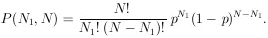The binomial distribution (14)

Note thatj=1N p(j, N) = [p + (1 - p)]N = 1. The factorials correct for the fact that we are not interested in the order in which the events occurred. For a given experimental result of N1 out of N events in Class 1, the likelihood function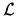(p) is then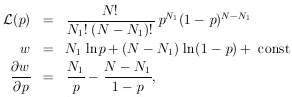(15)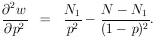(16)

From Eq. (15) we have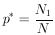(17)

From (16) and (17):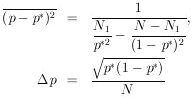(18)

The results, Eqs. (17) and (18), also happen to be the same as those using direct probability. Then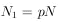and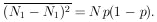Example 4

In Example 1 on the µ-e decay angular distribution we found that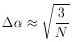is the error on the asymmetry parameter. Suppose that the individual cosine, xi, of each event is not known. In this problem all we know is the number up vs. the number down. What then is? Let p be the probability of a decay in the up hemisphere; then we have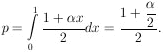By Eq. (18),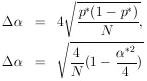For smallthis is= sqrt[4 / N] as compared to sqrt[3 / N] when the full information is used.